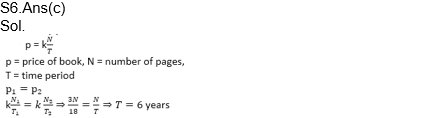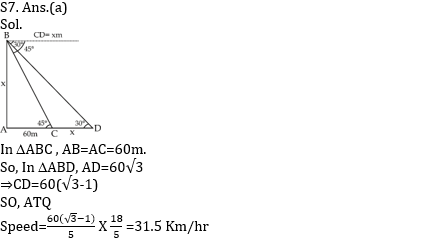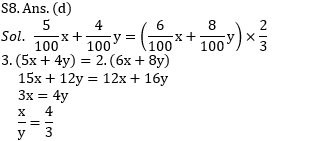# Mathematics Quiz For RRB NTPC : 7th December

Q1. The selling price of 10 oranges is the cost price of 13 oranges. then the profit percentage is :
(a) 30%
(b) 10%
(c) 13%
(d) 3%

Q1. Ans. (a)
Sol.
Profit percentage = (13-10)×100/10 = 30%

Q2. A circle of radius 8 cm has its radius decreased by 2 cm. What is the approximate percentage decrease in the area?
(a) 25%
(b) 50%
(c) 38%
(d) 43.75%

Q2. Ans. (b)
Sol.
Original area of the circle = π (8)2 = 64 π
New area of the circle = π (6)2 = 36 π
% decrease in area = 64 π – 36 π / 64 π x 100 = 43.75%

Q3. The sum of three consecutive odd numbers is 20 more than the smallest number. What is the middle number?
(a) 7
(b) 9
(c) 11
(d) 5

Q3. Ans. (b)
Sol.
Let the numbers be x, x + 2 and x + 4. Then
x + (x + 2) + (x + 4) = x + 20
x = 7
So the middle number is 9.

Q4. A fruit seller had some apples. He sells 40% apples and still has 420 apples. Originally, he had:
(a) 600 Apples
(b) 700 Apples
(c) 500 Apples
(d) None of these

Q4. Ans. (b)
Sol.
Suppose originally he had x apples.
Then, (100 – 40)% of x = 420.
60/100 × x = 420
x = (420 x 100)/60 = 700.

Q5. Two trains 140 m and 160 m long run at the speed of 60 km/hr and 40 km/hr respectively in opposite directions on parallel tracks. The time (in seconds) which they take to cross each other, is:
(a) 10.8 sec
(b) 9.5 sec
(c) 7.4 sec
(d) 8.9 sec

Q5. Ans. (a)
Sol.
Relative speed = (60 + 40) km/hr = 100×5/18 = 250/9 m/ sec.
Distance covered in crossing each other = (140 + 160) m = 300 m.
Required time = 300×9/250 = 54/5 = 10.8 sec.

Q6. When a commodity is sold for Rs 34.80, there is a loss of 25%. What is the cost price of the commodity?
(a) 46.40
(b) 43
(c) 26.10
(d) 43.20Q7. The two numbers are in the ratio 2:3 and their product is 54 the sum of the numbers is?
(a) 15
(b) 5
(c) 9
(d) 6Q8. In how many years a sum of money will triple itself at 8% per annum simple interest.
(a) 25 years
(b) 37.5 years
(c) 20 years
(d) 30 yearsQ9. In how much time a train of length 120 m running at 45km/hr can cross a platform of length 230 m?
(a) 36 sec
(b) 28 sec
(c) 14 sec
(d) 18 sec

Q9. Ans. (b)
Sol.
Crossing time = (120 + 230)/(45 * 5/18) = 28sec

Q10. What is 6/11 of 25% of 10% of 4400?
(a) 11
(b) 66
(c) 60
(d) 36

Q10. Ans. (c)
Sol.
6/11 of 25% of 10% 4400 = 6/11 * 25/100 * 10/100 * 4400 = 60

Important Links for RRB NTPC Recruitment 2019
RRB NTPC Previous year Cut Off | 1st & 2nd Stage Examination
RRB NTPC Recruitment 2019: Check FAQs
RRB NTPC Exam Pattern 2019 – Check Here
RRB NTPC Previous Year Exam Analysis
RRB NTPC Exam Syllabus×

Thank You, Your details have been submitted we will get back to you.Join India largest learning distination

What You Will get ?

•Job Alerts
•Daily Quizes
•Subject-Wise Quizes
•Current Affairs
•previous year question papers
•Doubt Solving session

ORJoin India largest learning distination

What You Will get ?

•Job Alerts
•Daily Quizes
•Subject-Wise Quizes
•Current Affairs
•previous year question papers
•Doubt Solving session

ORJoin India largest learning distination

What You Will get ?

•Job Alerts
•Daily Quizes
•Subject-Wise Quizes
•Current Affairs
•previous year question papers
•Doubt Solving session

Enter the email address associated with your account, and we'll email you an OTP to verify it's you.Join India largest learning distination

What You Will get ?

•Job Alerts
•Daily Quizes
•Subject-Wise Quizes
•Current Affairs
•previous year question papers
•Doubt Solving session

## Enter OTP

Please enter the OTP sent to
/6

Did not recive OTP?

Resend in 60sJoin India largest learning distination

What You Will get ?

•Job Alerts
•Daily Quizes
•Subject-Wise Quizes
•Current Affairs
•previous year question papers
•Doubt Solving sessionJoin India largest learning distination

What You Will get ?

•Job Alerts
•Daily Quizes
•Subject-Wise Quizes
•Current Affairs
•previous year question papers
•Doubt Solving session

## Almost there

+91Join India largest learning distination

What You Will get ?

•Job Alerts
•Daily Quizes
•Subject-Wise Quizes
•Current Affairs
•previous year question papers
•Doubt Solving session

## Enter OTP

Please enter the OTP sent to Edit Number

Did not recive OTP?

Resend 60

## By skipping this step you will not recieve any free content avalaible on adda247, also you will miss onto notification and job alerts

Are you sure you want to skip this step?

## By skipping this step you will not recieve any free content avalaible on adda247, also you will miss onto notification and job alerts

Are you sure you want to skip this step?